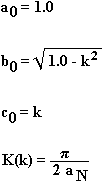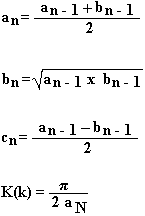# Elliptical Integrals of the First Kind Calculator

This calculator finds the elliptical integral K(k) and shows each successive step until the final result is obtained. This method of calculation is used in our Coplanar Waveguide With Ground Calculator and the equations used are shown below.

 Enter value k: Iteration (n) an bn cn K(k) at iteration N = n 0 1 2 3 4 5 6 7 K(k) =  Solution

This calculator uses JavaScript and will function in most modern browsers. For more information see About our calculators

These recursive equations were originally given for use in calculating the inductance of helical coils and are taken from Miller, H Craig, "Inductance Equation for a Single-Layer Circular Coil" Proceedings of the IEEE, Vol. 75, No 2, February 1987, pp. 256-257.

The first iteration (n0) is calculated with the following equations.Each successive iteration is then calculated with the following equations until cn = 0 is within the required accuracy.When cn = zero the value for K(k) is the required answer.

This calculator is provided free by Chemandy Electronics in order to promote the FLEXI-BOX